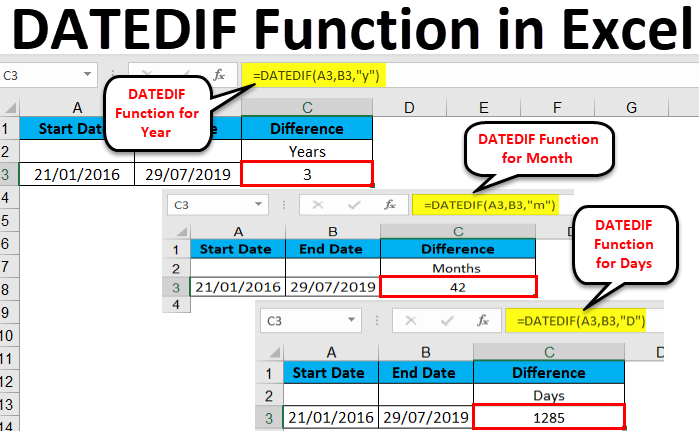# How To Use VBA DateDiff Function In Excel

Oct 23, 2020 • edited Oct 24, 2020The DateDiff function in Excel VBA can be used to get the number of days between two dates.

Place a command button on your worksheet and add the following code lines:

Dim firstDate As Date, secondDate As Date, n As Integer firstDate = DateValue(“Jan 19, 2020”) secondDate = DateValue(“Feb 25, 2020”) n = DateDiff(“d”, firstDate, secondDate) MsgBox n

Explanation: first, we declare two dates. Next, we initialize the two dates using the DateValue function. The DateDiff function has three arguments. Fill in “d” for the first argument since we want the number of days between two dates. Finally, we use a MsgBox to display the number of days between the two dates.

Result when you click the command button on the sheet:Change “d” to “ww” to get the number of weeks between two dates. Place your cursor on DateDiff in the Visual Basic Editor and click F1 for help on the other interval specifiers.

#Tutorial#How To#VBA#Date and Time

How To Use VBA Custom Average Function In Excel

How To Use VBA Debugging In Excel